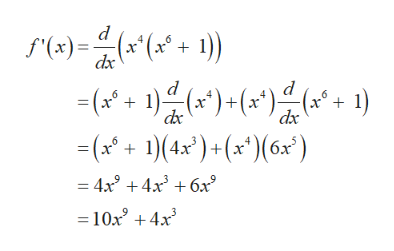Find the derivative of the function in the following two ways.x4(x6 + 1)(a) Find the derivative of the function using the Product Rule. [x4(x6 + 1)] =   (b) Find the derivative of the function by multiplying out the function and using the Power Rule. [x4(x6 + 1)] =

Question

Find the derivative of the function in the following two ways.

x4(x6 + 1)
(a) Find the derivative of the function using the Product Rule.
[x4(x6 + 1)] =

(b) Find the derivative of the function by multiplying out the function and using the Power Rule.
[x4(x6 + 1)] =

Step 1

Calculation:

Let f(x) = x4(x6 + 1)

(a)

Compute the derivative of f(x) usi...help_outlineImage Transcriptionclosed (x"(x* + 1) f'(x) dx -(1())(1) ) + (x*); (a21 dx -(14x)+ (x ) 6x ) = 4x3 4x3 6x 10x +4x fullscreen

Want to see the full answer?

See Solution

Want to see this answer and more?

Our solutions are written by experts, many with advanced degrees, and available 24/7

See Solution
Tagged in

Other